## Friday, November 14, 2008

### Irodov Problem 1.249As soon as the disc is placed flatly on the floor, each point on the disc starts to experience a force of friction dF that opposes its motion relative to the floor. While the friction force dF experienced at each point on the disc is the same (since the problem states that the disc applies equal pressure from all points), the resistive torque r x dF generated by the friction force depends on its distance from the center of the disc. Points of the disc that are far from the center generate more resistive torque than those that are closer to the center.

Let us consider an infinitesimally small section of the disc that is at a distance r from the center and has a thickness dr and at an angle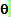and subtends an angle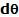from the center of the disc. The total area of this infinitesimally small part of the disc is given by. The mass dm of this small area of the disc is given by the area spanned by the disc multiplied by the mass per unit area of the disc,There are two forces acting on this small piece in the vertical direction the normal reaction from the surface dN and the force of gravity (dm)g acting downwards, and its acceleration in this direction is 0. In other words,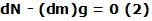The force of friction dF experienced by this piece will be equal to kdN and will act tangentially to the circle of radius r since its motion is along a cirlce of radius r as the disc spins. The resistive torque experienced by the piece will now be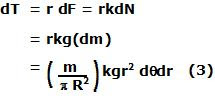The net torque is given by,Since the moment of inertia of the disc about its axis of rotation is given by, the angular retardation (deceleration) experienced by the disc is given by,Given that the disc was originally spun at an angular velocity of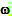, the time taken by it to stop will be given by,